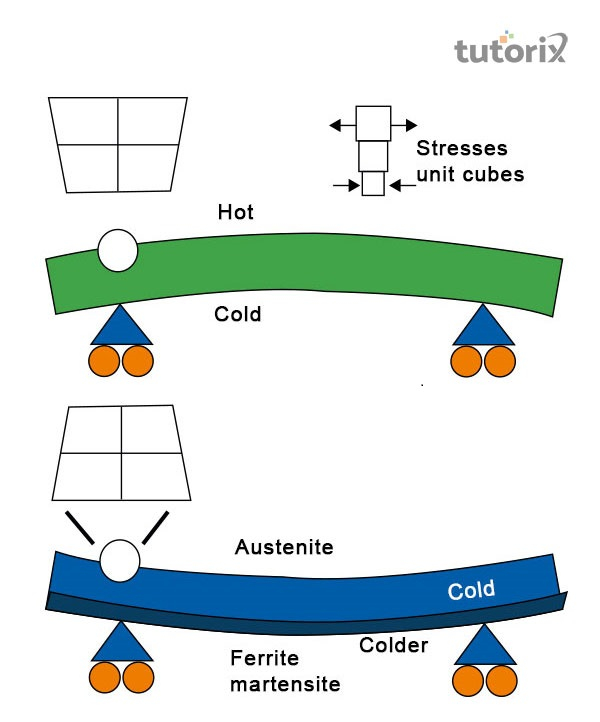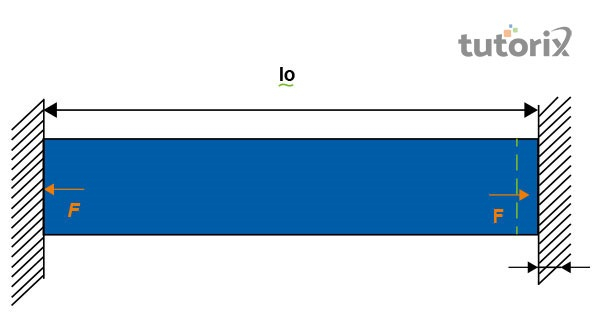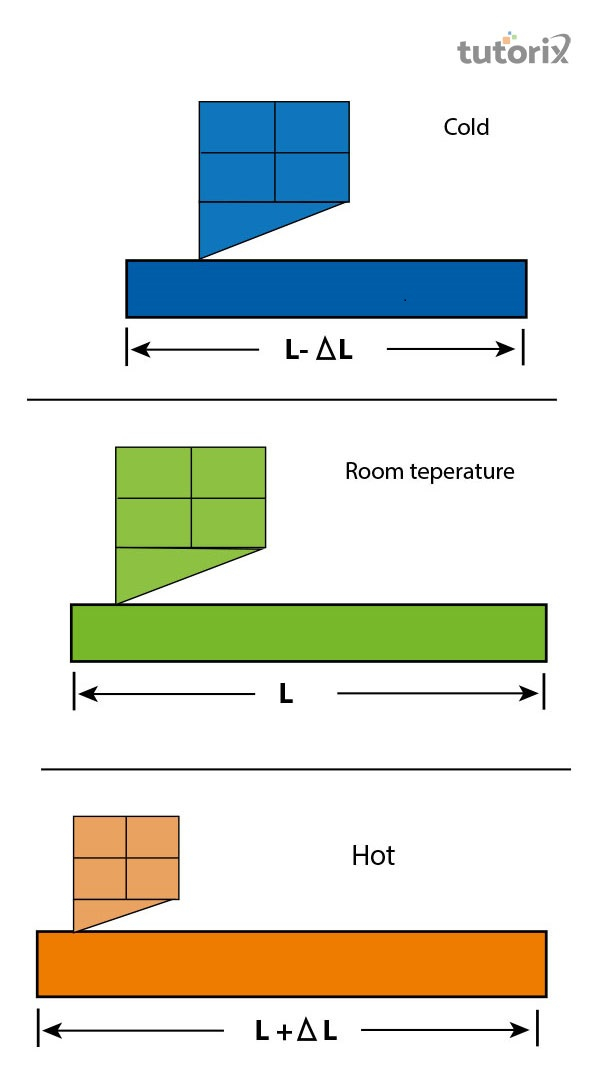# Thermal Stress

## Introduction

Each member of a metallic structure is impacted by thermal expansion. The thermal deformation is generated as a result of changes in the surrounding temperature. Being impacted by an external force, a body may get deformed.

The range and amount of deformation very much depend on different types of molecular attraction of a particular body. As a result of deformation, each significant internal force of a body plays an important role in bringing back this state in its initial state.

## What is thermal stress?

Stress is a significant force that acts per unit area. At a certain time when the applied force is related to temperature, this stress is known as thermal stress. The magnitude of a particular internal force increases as the range of deformation rises side by side (Zainuddin et al. 2020). Thermal stress is only observed at a time in which the particles of an object expand as affected by temperature changes.Figure 1: Thermal stress and strain

## Thermal stress formula

The values of deformation, related to thermal stress are described with the help of a particular formula.

$$\mathrm{\delta_t\:=\:\alpha\:*\:L\:*\:(T\:-\:T_0)}$$

In this formula, 𝛿t denotes the deformation range of a particular structural member. This deformation is expected to be a result of changes in temperature. The α represents the temperature coefficient of structural expansion. This is an important material property that is measured by using the unit Kelvin (K). The L in this formula is used to present the value of the original length of a structural member (Novak et al. 2021). Feet and meters are used to present the value of L. The final temperature, measured in Celsius and Kelvin is presented with T. T0 represents the value of the initial temperature. For an international system and also for the English system, this value is presented in K or C0.

The formula for calculating thermal stress is important and presented as follows. In the case of a thermal conducting rod, a change is seen in the length of this rod (Keulen et al. 2018). This change is proportional to the portion and amount of provided temperature. The coefficient of thermal expansion is expressed with a particular equation.

$$\mathrm{\delta_T\:=\:L\alpha(T_f\:-\:T_i)}$$

$$\mathrm{\delta_T\:=\:L \alpha \Delta T}$$

In this formula, L represents the value of length. This is expressed with the unit meter. Ti is the value of initial temperature that is expressed by unit 0C. The value of Tf is known as the value of final temperature, expressed in 0C. The changes in temperature are denoted by $\mathrm{\delta_T}$. In 0C, this value is equal to (Tf - Ti). The coefficient value of thermal expansion is presented by α and its unit is m/m0C.

## Causes of thermal stress

Based on the principles of thermodynamics, thermal stress is an important mechanical process that deals with changes in the internal temperature of a particular object. The main cause of thermal stress is the rise and fall of temperature in external temperature (Chang et al. 2019). As a result of thermal shock as well, thermal stress results in cracking and even breaking an object.Figure 2: Thermal stress

## Applications of thermal stress

The most basic and popular application of thermal stress is found in thermostats. The word thermos is used to present temperature and the word stat is used to present static. The thermostat is a device, used to regulate and maintain a constant temperature. Thermostats are also used in some of the constructing devices such as air conditioners and iron boxes (Shittu et al. 2019). Another important application of thermal stress lies in the preparation of bimetallic strips of an iron box. Each thermostat possesses an iron box. Two different metals are used in forming a bimetallic strip.

## Working of thermal stressFigure 3: Working of thermal stress

The working procedure of thermal stress changes according to the changes in the surrounding temperature. At room temperature, a contact point remains in physical contact that is attached to bimetallic strips. The moment of temperature crosses the limit by extreme heating. As a result, the bimetallic strip starts to bend. The changes in surrounding temperature are the main reason behind this (McClanahan et al. 2020). These changes are expressed by identifying the changes in the length of the structure. At room temperature, the length of an object is presented as L which becomes $\mathrm{L\: -\:\Delta L}$ at cold temperature. This length is changed into $\mathrm{L\: + \:\Delta L}$ when the temperature changes to hot.

## Conclusion

The stability and strength of a structure are highly affected if proper checking is not done regarding thermal stress. Negligence in evaluating the power of thermal stress can result in creating cracks and even cause breakage to various kinds of structural components. After heating the glass at a very high temperature for a certain time and then immediately dipping it into cold water, the glass breaks into pieces. Immediate changes in temperature lead the glass to lose its grip and break. The fracture and crack in the glass are very different from each other as the impact of thermal stress is different in nature. This example is important to understand the effects of thermal stress on a structural body.

## FAQs

Q1. Which unit is used to present thermal stress?

Ans. Force per unit area is used to symbolize stress that is necessary to deform a particular object. In the CGS unit, the stress is expressed in dyne $\mathrm{cm^{-2}}$. The SI unit, $\mathrm{Nm^{-2}}$ is used to express thermal stress.

Q2. What are the important thermals properties of a structure and material?

Ans. Thermal stress, heat capacity, thermal conductivity, and thermal expansion are significant properties of a material. The quantity of heat is dependent on this.

Q3. How heat capacity is related to thermal stress?

Ans. Thermal stress is a result of changes in external and internal temperature. The quantity of heat that is needed to cause changes in temperature is known as heat capacity.

Q4. Which formula is used to calculate the value of heat capacity?

Ans. The formula "Q = mc AT" is used to extract the value of heat capacity. This value helps in measuring the value of thermal stress as well.

Updated on: 13-Oct-2022

271 Views